# Physics Experiments

## Physics Experiments

NeuLog logger sensors help us explore and understand the laws of physics that are the very fabric of the universe around us.  The laws of mechanics, sound, light, heat, electricity, magnetism are only some of the phenomena that the logger sensors measure, display and safely store.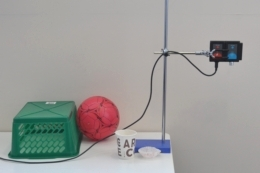Falling Objects P-1 ver. 3.6.12 Motion logger sensor Study the motion of falling objects. Learn about the effect of air resistance force. Study motion parameters of a bouncing ball.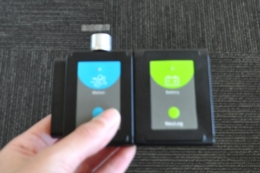Walking Analysis P-2 ver. 3.10.7 Motion logger sensor Learn about motion parameters. Study the motion parameters of walking.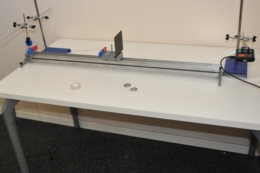Motion Parameters of a Moving Cart P-3 ver. 3.6.9 Motion logger sensor Learn about motion parameters. Study the relationship between position, velocity and acceleration of a moving cart.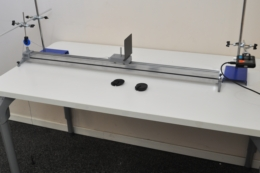Newton’s Second Law P-4 ver. 3.14.7 Motion logger sensor Study the relationship between force, mass and acceleration according to Newton’s second law. Use different masses and examine the results.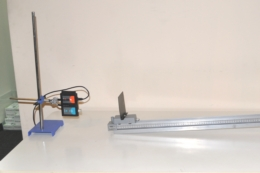Motion of a Cart in an Inclined Plane P-5 ver. 3.0.2 Motion logger sensor Learn about the four motion equations. Study the motion of a cart on an inclined plane. Study motion with constant acceleration.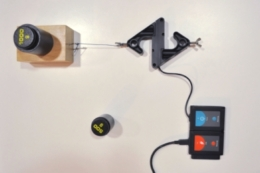Friction Force P-6 ver. 3.4.6 Force logger sensor Learn about the relationship between friction force, normal force and friction coefficient. Observe changes in the friction force within different surfaces and different masses. Calculate the friction coefficients (static and kinetic) in these different conditions.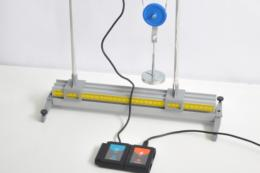Pulley System P-8 ver. 3.3.5 Force logger sensor Learn about pulley systems: fixed, movable and compound pulleys. Measure the effort force and the load force. Measure the distance the force sensor has to be pulled in order to move the load 10 cm and calculate the velocity ratio. Calculate the mechanical advantage of each system and find the relationship between the mechanical advantage and the velocity ratio.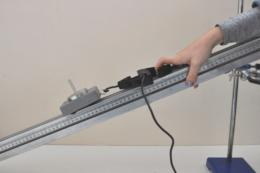An Inclined Plane P-9 ver. 3.0.5 Force logger sensor Understand the principles of forces on an inclined plane. Measure the parallel component of the gravitational force and compare it to the calculated force. Compare the forces within different angles and masses.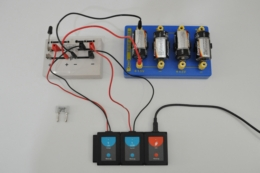Ohm’s Law P-10 ver. 3.4.7 Voltage logger sensor Current logger sensor Study the relationship between the voltage applied to a given resistor and the intensity of the current running through it.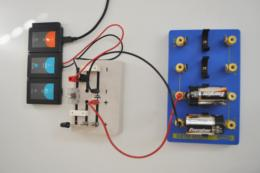How Incandescent Light Bulbs Work? P-11 ver. 3.4.4 Current logger sensor Light logger sensor Learn how incandescent light bulbs work. Measure the time it takes for a light bulb to turn on from the moment current flows. Investigate the effect of heating the filament on the current.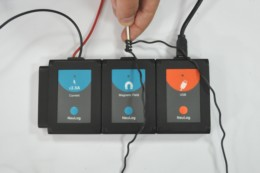Basic Electromagnetism P-16 ver. 3.3.4 Current logger sensor Magnetic field logger sensor Learn about electromagnets. Build an electromagnet with a nail, a wire and additional electrical elements. Investigate how the number of winds affect the magnetic field of the electromagnet.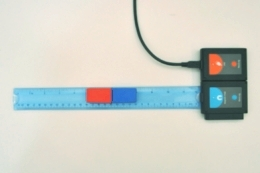Magnetic Field Strength P-17 ver. 3.4.5 Magnetic field logger sensor Learn about basic properties of magnets. Study the relationship between magnetic field strength and the distance from the magnet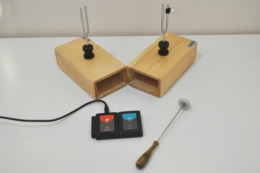Sound Beats P-22 ver. 3.3.11 Sound logger sensor Observe the beats of sound waves produced by two tuning forks on resonance boxes. Understand the concept of wave interference.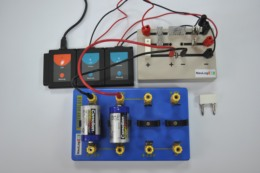Circuits and Series Resistance P-24 ver. 3.4.4 Voltage logger sensor Current logger sensor Study the relationship between the voltage applied to a given resistor and the intensity of the current running through it.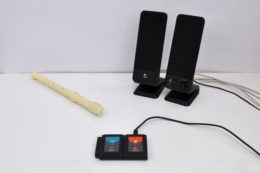Exploring Sound P-26 ver. 3.0.9 Sound logger sensor Learn how to measure different intensities of sound. Understand the meaning of sound units (dB). Get a feeling of what dangerous high intensities of sound mean.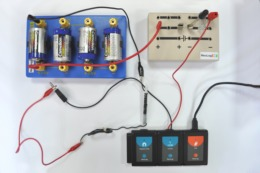Current Variations and Generation of a Magnetic Field P-27 ver. 3.0.4 Current logger sensor Magnetic field logger sensor Learn about electromagnets. Build an electromagnet with a nail, a wire and an electrical circuit device. Investigate how the current affects the magnetic field of the electromagnet.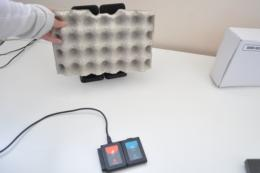Sound Isolation P-29 ver. 3.0.6 Sound logger sensor Observe through which materials sound travels better. Understand the concept of sound isolation.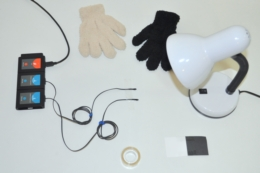Light Absorption and Heat P-31 ver. 3.3.13 Two Surface temperature logger sensors Understand the concept of color. Demonstrate the effect of color on light absorption and the conversion of that light into heat, using black and white papers. Measure body surface temperature while being covered with black or white gloves.Sound waves P-33 ver. 3.4.7 Sound logger sensor Study sound waves produced by a tuning fork on a resonance box. Learn about period and frequency of sound waves. Understand how the length of the tuning fork affects its sound.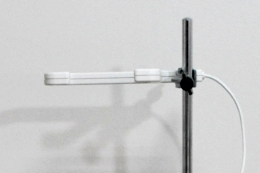Velocity of Free Falling Objects P-35 ver. 3.0.5 Photo gate logger sensor Measure the velocity of falling objects at a minimal air resistance. Examine whether two objects with different masses have the same velocity while falling.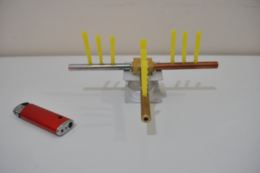Thermal Conductivity P-38 ver. 3.0.4 Temperature logger sensor Understand the concept of thermal conductivity. Investigate the thermal conductivity of different metals using qualitative and quantitative methods.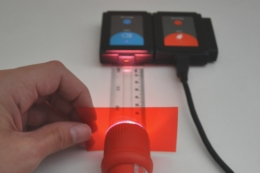Colors of Light P-40 ver. 3.0.5 Light logger sensor Learn what happens to colored light, when it passes through color filters. Investigate the additive color theory. Observe a stereoscopic image.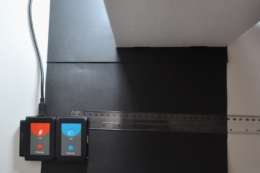Light and Shadow P-41 ver. 3.0.7 Light logger sensor Understand the principles of light and shadow. Measure the intensity of light at the unblocked region, the penumbra region (partly blocked), and the umbra region (fully blocked). Investigate different parameters affecting a casted shadow.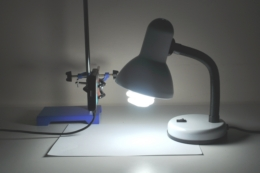Light and Dark Colors P-42 ver. 3.0.7 Light logger sensor Learn about light reflected from light and dark objects, and the amount of light they absorb. Understand the way we perceive different colors and shades.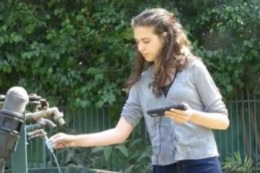Flowing Water P-43 ver. 3.0.2 Flow logger sensor Investigate water velocity in different positions of a falling stream.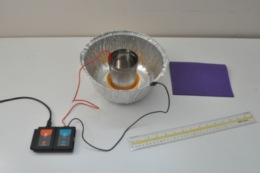Static Electricity P-44 ver. 3.0.4 Charge logger sensor Learn about static electricity and how it is created. produce static electricity by rubbing different materials against each other, in order to measure it.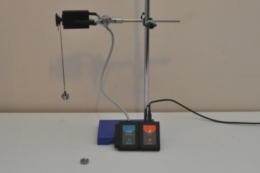Simple Pendulum P-46 ver. 3.0.4 Rotary motion logger sensor Learn about the period and frequency of a simple pendulum. Understand the difference between a simple pendulum and a real life pendulum. Examine the effects of length and mass on the pendulum’s period.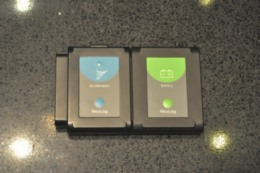Acceleration of an Elevator P-47 ver. 3.0.4 Acceleration logger sensor Learn about acceleration and the principles of an acceleration sensor (accelerometer). Analyze the acceleration of a moving elevator or vehicle.Impulse and Momentum P-48 ver. 3.0.4 Force plate logger sensor Learn about the relation between impulse and momentum. Examine this relation by bouncing a ball on a force plate logger sensor and measuring the applied force. Calculate impulse and change in momentum, then compare these values.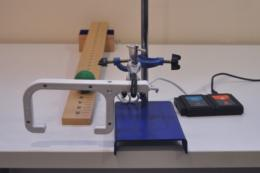Horizontal Launched Projectile Motion P-49 ver. 3.0.5 Photo gate logger sensor Understand the concepts of projectile motion and trajectories. Predict the landing point of a ball by measuring its initial horizontal velocity. Generate different velocities and observe the compatible motion pattern.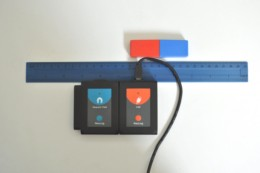Magnetic Field P-52 ver. 3.0.4 Magnetic field logger sensor Learn about basic properties of magnets. Study the magnetic field around a bar magnet through a magnetic field sensor.Partial Vacuum P-53 ver. 3.0.4 Pressure logger sensor Study the concept of vacuum and partial vacuum. Investigate how pumping out air from a box affect the pressure inside. Investigate how the change of pressure affects a balloon and a marshmallow that are in the box.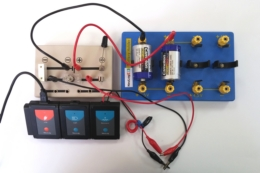Resistance of a Light Bulb P-54 ver. 3.0.2 Light logger sensor Resistance logger sensor Learn how incandescent light bulbs work. investigate the effect of heating the bulb’s filament on the resistance

#### Upcoming Experiments:

Levers,  Sound Rate, Light intensity and distance, An Electric Current in Closed and Opened Circuits.